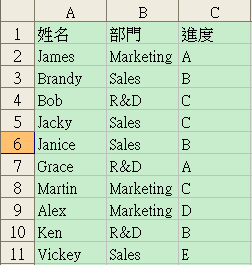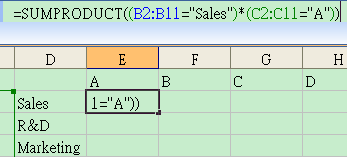Excel 多條件變數 countif 函數 sumproduct and or - 通達人驛站

# Excel 多條件變數 countif 函數 sumproduct and or

#### 在 SUMPRODUCT 內使用 and=SUMPRODUCT((B2:B11="Sales")*(C2:C11="A"))SUMPRODUCT 內的「*」就是 and 的意思。

#### 在 SUMPRODUCT 內使用 or

=SUMPRODUCT((B2:B11="R&D")*((C2:C11="A")+(C2:C11="B"))

SUMPRODUCT 內的「+」就是 or 的意思。

#### 將 SUMPRODUCT True/False 陣列轉換為 1/0 陣列

=SUMPRODUCT(--(B2:B11="Sales"),--(C2:C11="A"))

=SUMPRODUCT(--(B2:B11="R&D"),--((C2:C11="A")+(C2:C11="B"))

=ARRAYFORMULA(SUMPRODUCT((B2:B11="Sales")*(C2:C11="A")))

#### 注意事項

1. mrexcel -《COUNTIF with two Variables?
﻿﻿